Processing ......FreeComputerBooks.com Links to Free Computer, Mathematics, Technical Books all over the World

A Problem Course in Mathematical Logic
Top Free Machine Learning Books 🌠 - 100% Free or Open Source!
• Title A Problem Course in Mathematical Logic
• Authors Stefan Bilaniuk
• Publisher: Orange Grove Texts Plus (September 24, 2009)
• Paperback: 166 pages
• eBook: In LaTeX, PDF, and PostScript formats
• Language: English
• ISBN-10: 1616100060
• ISBN-13: 978-1616100063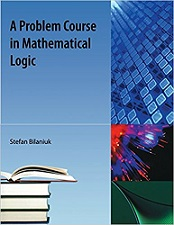Book Description

A Problem Course in Mathematical Logic is intended to serve as the text for an introduction to mathematical logic for undergraduates with some mathematical sophistication. It supplies definitions, statements of results, and problems, along with some explanations, examples, and hints. The idea is for the students, individually or in groups, to learn the material by solving the problems and proving the results for themselves. The book should do as the text for a course taught using the modified Moore-method.

The material and its presentation are pretty stripped-down and it will probably be desirable for the instructor to supply further hints from time to time or to let the students consult other sources. Various concepts and and topics that are often covered in introductory mathematical logic or computability courses are given very short shrift or omitted entirely, among them normal forms, definability, and model theory.

Parts I and II, Propositional Logic and First-Order Logic respectively, cover the basics of these topics through the Soundness, Completeness, and Compactness Theorems, plus a little on applications of the Compactness Theorem. They could be used for a one-term course on these subjects. Part III, Computability, covers the basics of computability using Turing machines and recursive functions; it could be used as the basis of a one-term course. Part IV, Incompleteness, is concerned with proving the Godel Incompleteness Theorems. With the omission of some topics from Part III which are not needed to prove the results in Part IV, Parts III and IV could be used for a one-term course for students who know the contents of Part II already.

• N/A
Reviews, Ratings, and Recommendations: Related Book Categories: Read and Download Links:Similar Books:
•A Friendly Introduction to Mathematical Logic (Chris Leary)

In this user-friendly book, readers with no previous study in the field are introduced to the basics of model theory, proof theory, and computability theory, leading to rigorous proofs of Gödel's First and Second Incompleteness Theorems.

•Introduction to Mathematical Logic (Vilnis Detlovs, et al)

This book explores the principal topics of mathematical logic. It covers propositional logic, first-order logic, first-order number theory, axiomatic set theory, and the theory of computability. Discusses the major results of Gödel, Church, Kleene, Rosser, and Turing.

•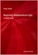Beginning Mathematical Logic: A Study Guide (Peter Smith)

It introduces the core topics and recommends the best books for studying these topics enjoyably and effectively. An invaluable resource both for those wanting to teach themselves new areas of logic and for those looking for supplementary reading.

•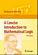A Concise Introduction to Mathematical Logic (W. Rautenberg)

This is a well-written introduction to the beautiful and coherent subject of mathematical logic. It contains classical material such as logical calculi, beginnings of model theory, and Goedel's incompleteness theorems, as well as some topics motivated by applications.

•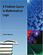A Problem Course in Mathematical Logic (Stefan Bilaniuk)

It is intended to serve as the text for an introduction to mathematical logic for undergraduates with some mathematical sophistication. It supplies definitions, statements of results, and problems, along with some explanations, examples, and hints.

•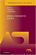Model-Theoretic Logics (Jon Barwise, et al)

This book brings together several directions of work in model theory between the late 1950s and early 1980s. It provides an introduction to the subject as a whole, as well as to the basic theory and examples. Many chapters can be read independently.

•Language, Proof and Logic (Jon Barwise, et al)

This book covers first-order language in a method appropriate for first and second courses in logic, and is specially useful to undergraduates of philosophy, computer science, mathematics, and linguistics.

•Logical Reasoning (Bradley H. Dowden)

The goal of this book is to improve your logical-reasoning skills. Your most important critical thinking skill is your skill at making judgments-not snap judgments that occur in the blink of an eye, but those that require careful reasoning.

•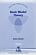Basic Model Theory (Kees Doets)

As the title indicates, this book introduces the reader to what is basic in model theory. A special feature is its use of the Ehrenfeucht game by which the reader is familiarised with the world of models.

•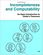Incompleteness and Computability: Gödel's Theorems

This book is an introduction to metamathematics and Gödel's Theorems. It covers recursive function theory, arithmetization of syntax, the first and second incompleteness theorem, models of arithmetic, second-order logic, and the lambda calculus.

Book Categories
 :All CategoriesTop Free BooksRecent BooksMiscellaneous BooksComputer EngineeringComputer LanguagesComputer ScienceData Science/DatabasesJava and Java EE (J2EE)Linux and UnixMathematicsMicrosoft and .NETMobile ComputingNetworking and CommunicationsSoftware EngineeringSpecial TopicsWeb Programming
Other Categories